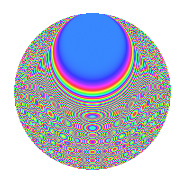# Properties

 Label 1008.2.dsLevel 1008 Weight 2 Character orbit ds Rep. character $$\chi_{1008}(269,\cdot)$$ Character field $$\Q(\zeta_{12})$$ Dimension 256 Sturm bound 384

# Related objects

## Defining parameters

 Level: $$N$$ = $$1008 = 2^{4} \cdot 3^{2} \cdot 7$$ Weight: $$k$$ = $$2$$ Character orbit: $$[\chi]$$ = 1008.ds (of order $$12$$ and degree $$4$$) Character conductor: $$\operatorname{cond}(\chi)$$ = $$336$$ Character field: $$\Q(\zeta_{12})$$ Sturm bound: $$384$$

## Dimensions

The following table gives the dimensions of various subspaces of $$M_{2}(1008, [\chi])$$.

Total New Old
Modular forms 800 256 544
Cusp forms 736 256 480
Eisenstein series 64 0 64

## Trace form

 $$256q + O(q^{10})$$ $$256q + 8q^{16} + 48q^{22} - 24q^{28} - 120q^{40} + 72q^{52} - 24q^{58} - 96q^{64} + 16q^{67} + 48q^{70} - 120q^{82} - 8q^{88} + 48q^{91} - 72q^{94} + O(q^{100})$$

## Decomposition of $$S_{2}^{\mathrm{new}}(1008, [\chi])$$ into newform subspaces

The newforms in this space have not yet been added to the LMFDB.

## Decomposition of $$S_{2}^{\mathrm{old}}(1008, [\chi])$$ into lower level spaces

$$S_{2}^{\mathrm{old}}(1008, [\chi]) \cong$$ $$S_{2}^{\mathrm{new}}(336, [\chi])$$$$^{\oplus 2}$$

## Hecke Characteristic Polynomials

There are no characteristic polynomials of Hecke operators in the database